# Intro to Mapping Class Groups, Teichmuller Spaces and Hyperbolic Surfaces.

October 8, 2017

I asked Kasra Rafi about the link between hyperbolic surfaces, Teichmüller spaces and Mapping Class Groups with the goal of getting an intuitive overview of these concepts. During the span of several office hours, he generously replied with a beautiful torus story linking the three, which I adapt and fill in below.

## Quick recap of definitions

Hyperbolic surface: A topological surface endowed with a hyperbolic metric such that the space has constant negative curvature. Recall from differential geometry that for Riemann surface with metric $ds$ where $ds^2 = g_1 dx_1^2 + g_2 dx_2^2$, the curvature is given by $K = -\frac{1}{\sqrt{g_1 g_2}}\left(\frac{\partial}{\partial x_1}\left(\frac{1}{\sqrt{g_1}} \frac{\partial \sqrt{g_2}}{\partial x_1}\right) + \frac{\partial}{\partial x_2}\left(\frac{1}{\sqrt{g_2}} \frac{\partial \sqrt{g_1}}{\partial x_2}\right) \right).$Adapted from Hyperbolic Geometry by Caroline Series and Wikipedia.

Teichmüller space: Let S be a compact surface. The Teichmüller space $\tau(S)$ is the set of isotopy classes of complex structures on $S$. By a complex structure on $S$, we mean a fixed homeomorphism $f: S \to X$, where $X$ is a metrized surface with distinguished curves. Equivalently, $\tau(S)$ is a space that parametrizes complex structures on $S$ up to the action of homeomorphisms that are isotopic to the identity. We may record the complex structure $f: S \to X$ by the triple $(S,X,f)$. The homeomorphism is referred to as a marking which has named curves, and either $X$ or $(X,f)$ can be referred to as a marked surface.Adapted from A Primer on Mapping Class Groups by Farb and Margalit and Wikipedia.

Mapping class group: The group of orientation-preserving homeomorphisms of a surface up to isotopy, where the group structure is inherited from functional composition of homeomorphisms. In other words, $\text{MCG}(S) = \text{Homeo}^+(S)/\text{Homeo}_0(S)$.where $\text{Homeo}_0(S)$ are homeomorphisms isotopic to the identity. The group multiplication of the mapping class group of $S$ gives it a natural action on the Teichmüller space of $S$ by composition of homeomorphisms.Adapted from A Primer on Mapping Class Groups by Farb and Margalit and Wikipedia.

At first glance, these seem tricky and confusing – let’s see what they mean in the context of one example.

## A humble beginning with lattices

Our story starts with a simple problem. We can define a lattice in $\mathbb{R}^2$ to be an injective homomorphism from $\mathbb{Z}^2 \to \mathbb{R}^2$.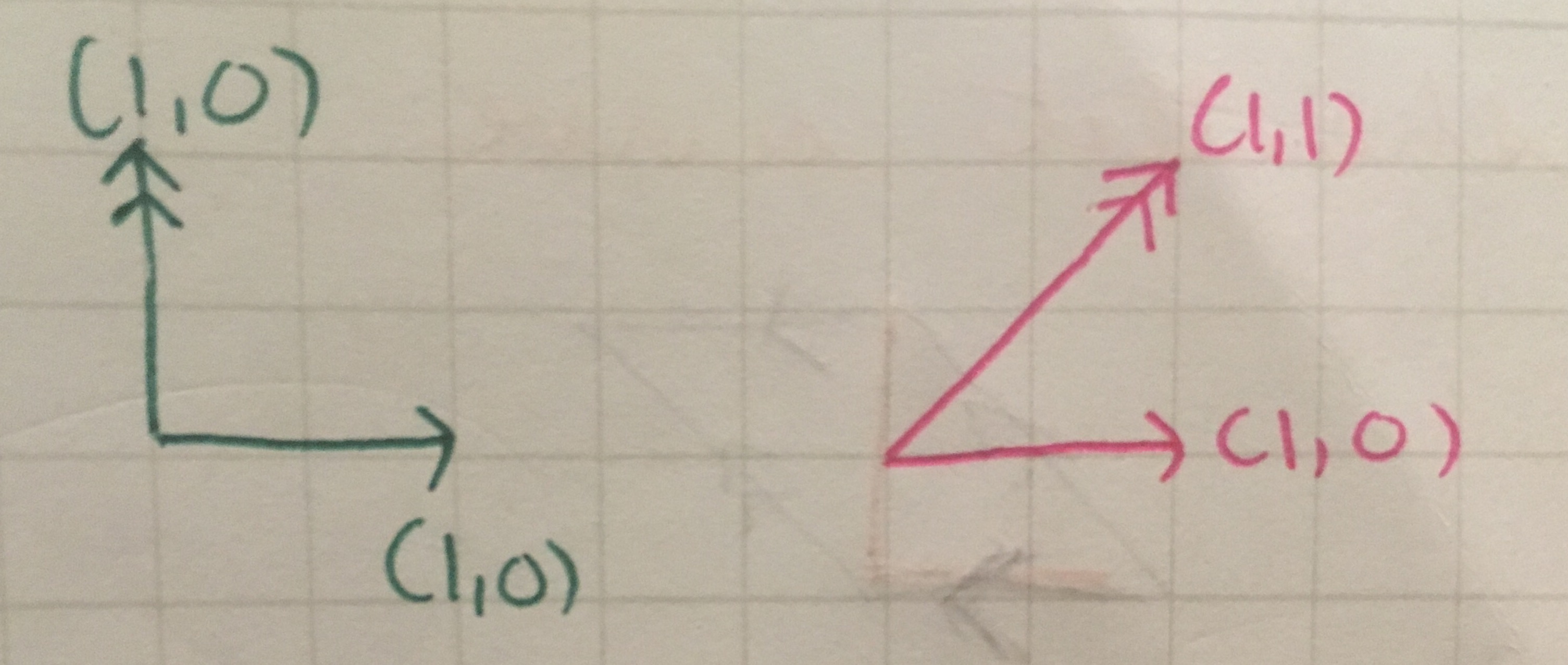We will be calling the two vectors defining a lattice the *basis* of the lattice. In the picture above, the left (green) represents the linear map $\{(1,0), (0,1)\} \mapsto \{(1,0), (0,1)\}$ and the right represents the linear map $\{(1,0), (0,1)\} \mapsto \{(1,0), (1,1)\}.$

Key idea: Up to what should these maps be equivalent? What is a good notion of equivalence to use?

One way to interpret this question is to define up to equivalence maps which have the same images. For example, the linear map ${(1,0), (0,1)} \mapsto {(1,0), (0,1)}$ and the linear map ${(1,0), (0,1)} \to {(1,0), (1,1)}$ have the same image, which is $\mathbb{Z}^2 \subset \mathbb{R}^2.$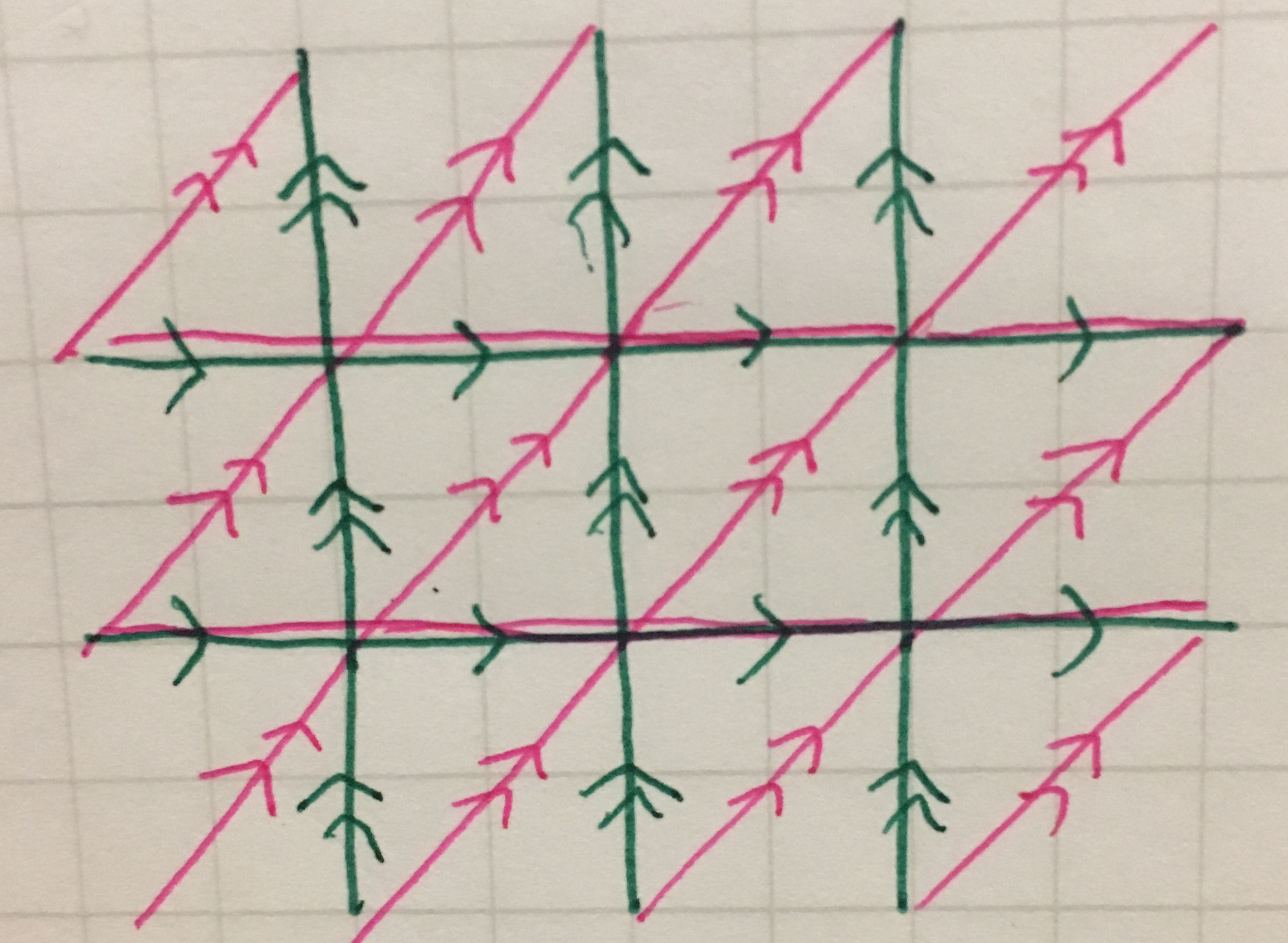Picture illustrating how the two maps have the same image as they span the same lattice points.

On the other hand, the linear map ${(1,0),(0,1)} \mapsto {(2,0), (1,2)}$ (in blue) does not have the same image as the other two (in brown). For example, the point $(1,1)$ (in pink) is not in the image of the linear map.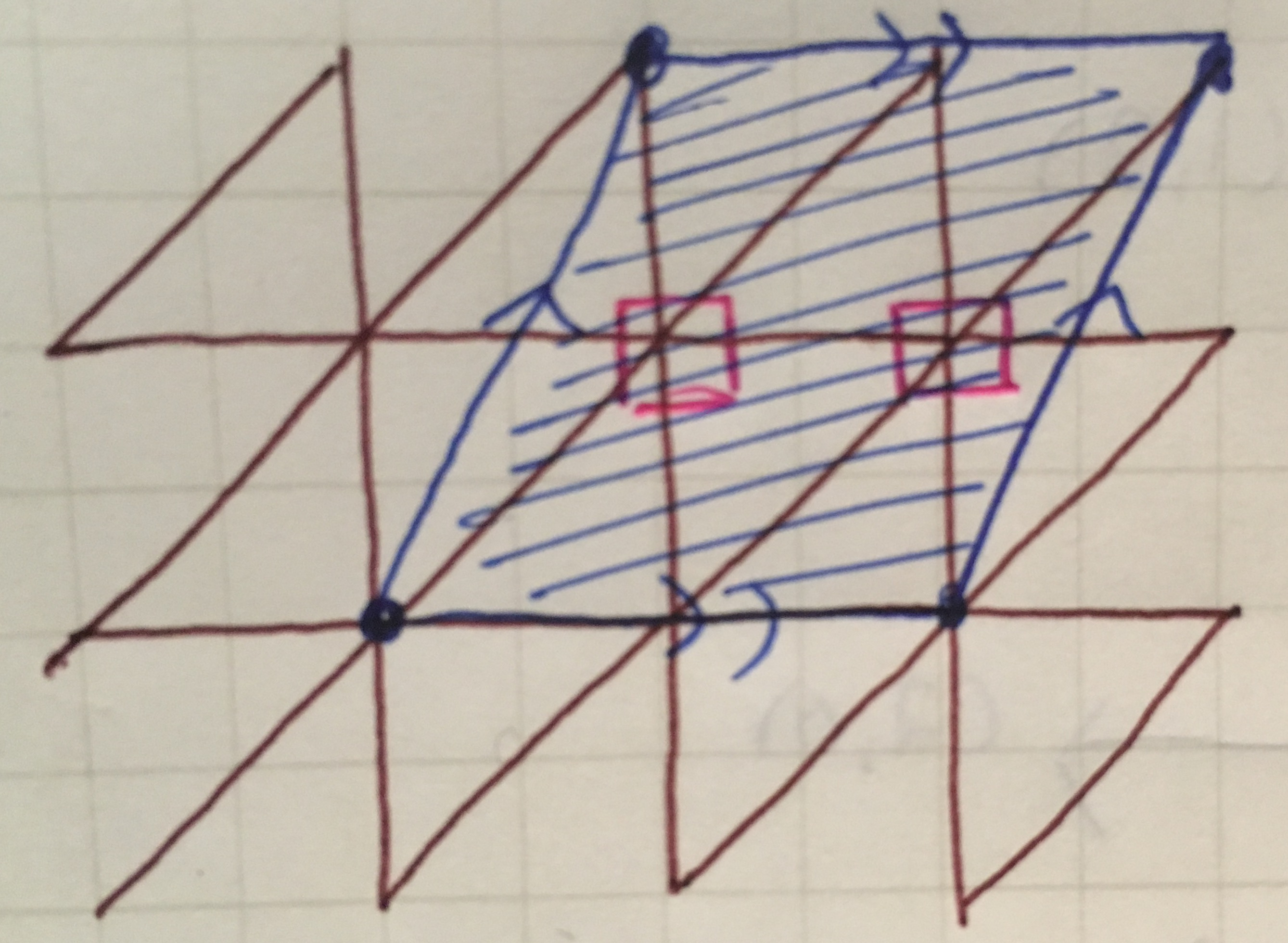In blue: the parallelogram formed by vectors $\{(2,0), (1,2)\}.$ In brown: the tesselation formed by the previous lattice. In pink: points not covered by the blue tesselation that are covered in the brown tesselation.

Already, we have a new notion of equivalence. Since we can choose to care about only the span of the image of the chosen basis elements for $\mathbb{Z}^2$, two maps could be equivalent under change of basis which preserves the span of the image. Thus, an equivalence of maps from $\mathbb{Z}^2 \to \mathbb{Z}^2 \subset \mathbb{R}^2$ must necessarily be area preserving (so that both base and height are $1$ in the domain as fractions are not allowed). IF we choose to preserve orientation,which we will (spoiler alert!) because we will want to associate each lattice with a marked torus, and a torus is an oriented surface. the group acting on these maps must be $\text{SL}_2(\mathbb{Z})$ the special linear group of two-dimensional integer matrices with determinant $1$.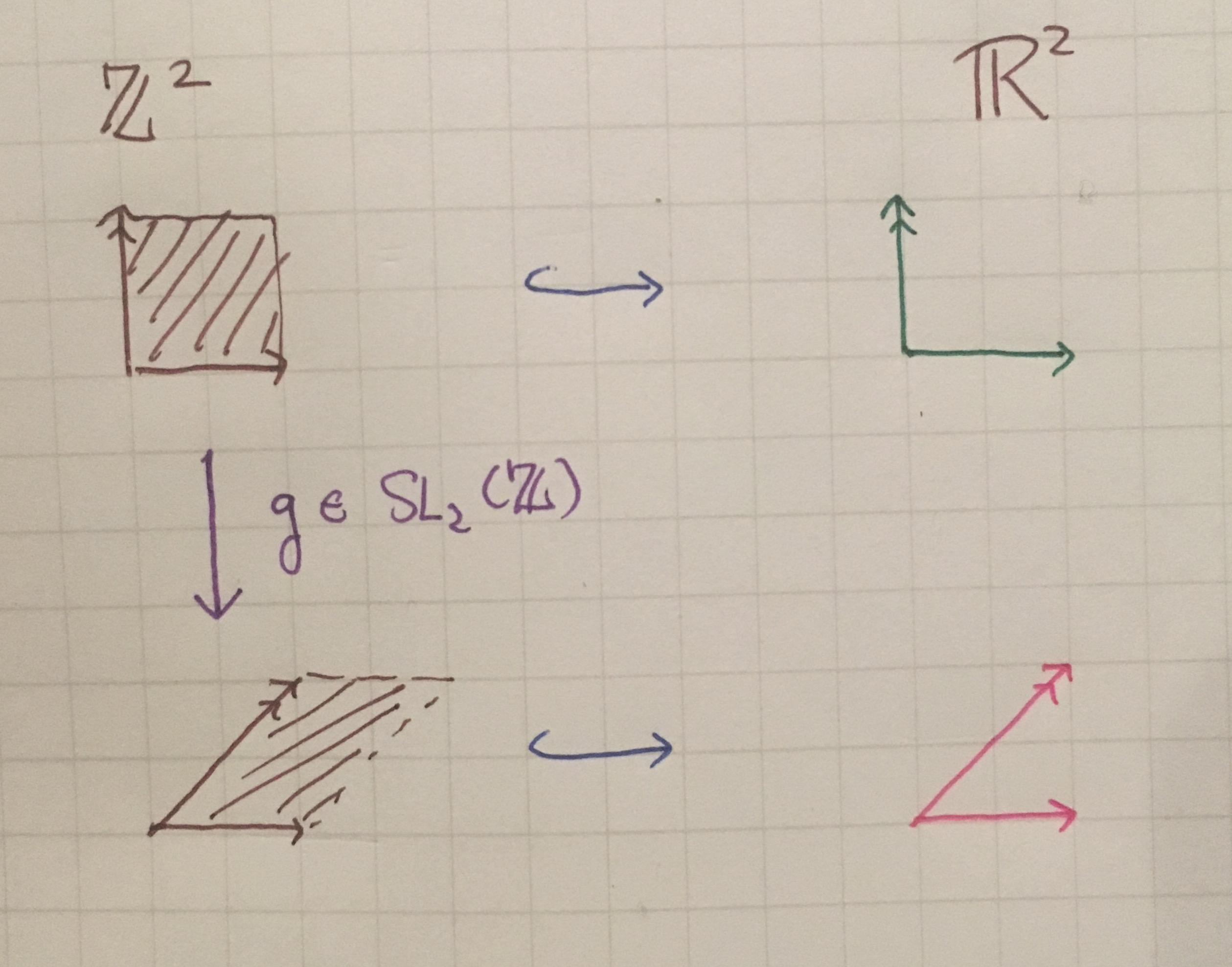Picture illustrating how $\text{SL}_2(\mathbb{Z})$ works on bases of $\mathbb{Z}^2$ the domain of the lattice maps. The map pictured here is the natural embedding (in blue) of $\mathbb{Z}^2$ into $\mathbb{R}^2,$ but it could be anything that is an injective linear map.

$\text{SL}_2(\mathbb{Z})$ is also known as the mapping class group of the torus. What torus? How do we get there?

## Torus time!

Since each parallelogram is exactly the same in a given lattice, it doesn’t matter which one we pick as a representative!

Under the assumption that all parallelograms in a given lattice is the same, we may identify the sides of the parallelograms accordingly. The resulting surface is a torus identified with a particular lattice $\mathbb{Z}^2 \to \mathbb{R}^2$.

Key idea: How do we transfer the equivalence relation between parallelograms onto its torus representative?

Unfortunately, genus 1 tori are all the same as topological spaces, which we write as $\mathbb{R}^2/\mathbb{Z}^2$. Since there is only one equivalence class for genus 1 tori under homeomorphisms, we will need finer notion of equivalence to get “interesting” equivalence classes”.

## Scale equivalence and $\text{SL}_2(\mathbb{R})$ action

Notice we have only talked about equivalence in the domain space $\mathbb{Z}^2$. In the image space, the completeness of $\mathbb{R}^2$ allows for richer equivalences.

Indeed, maybe instead of the image of maps being the distinguishing factor, we only care about the shape of the parallelograms in the lattice. That translates to equivalences up to scaling and rotation. Notice that both are features that we think of as invariant in a torus. These equivalences will lead us to the Teichmüller space of the torus. Notice

Let’s start with scaling and see what natural group action arises from it. If we decide to mod the lattices by a scaling factor, we may consider only the set of lattices whose parallelograms have area $1$.Imagine stretching your parallelogram freely until you get area $1$.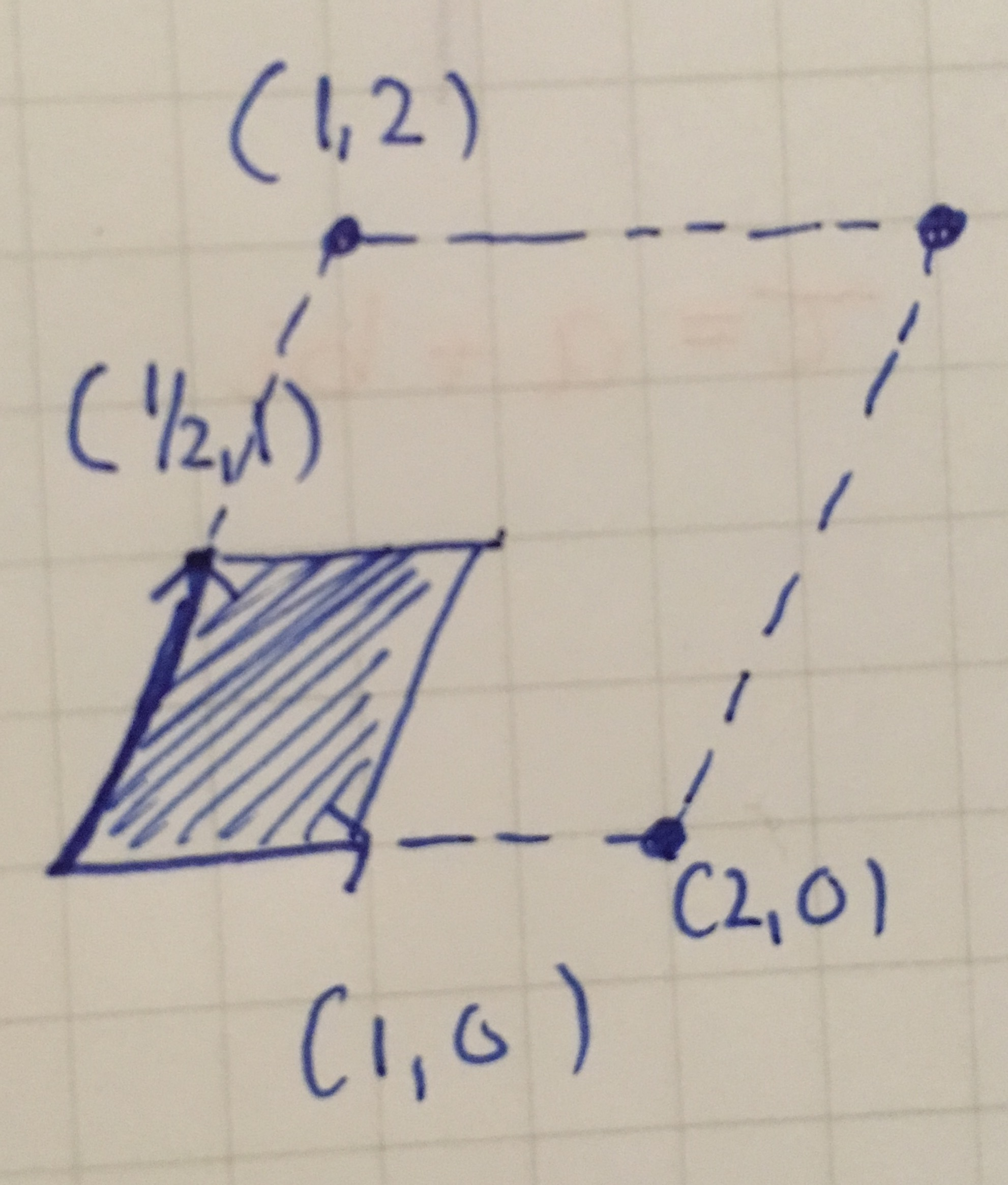Scaling our parallelogram to have area $1$.

Then, the group acting on parallelograms with area $1$ is $\text{SL}_2(\mathbb{R})$, the special linear group of two-dimensional real matrices with determinant $1$.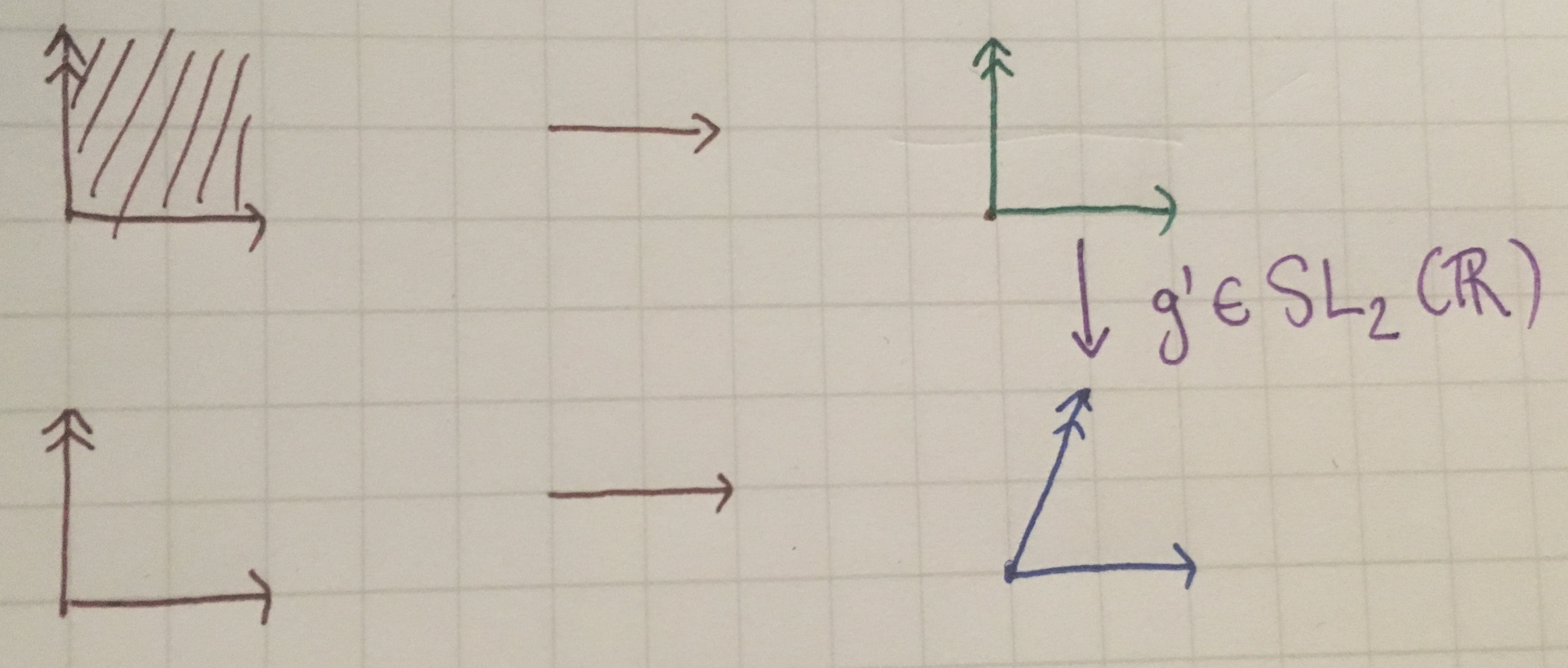Diagram showing where $\text{SL}_2(\mathbb{R})$ acts.

## Rotation and identification of torus with point in upper half-plane

For a given set of two vectors in $\mathbb{R}^2$, we may rotate a parallelograms such that the second basis vector is always in the upper half-plane. This does not change the torus we are looking at and neither does the scaling.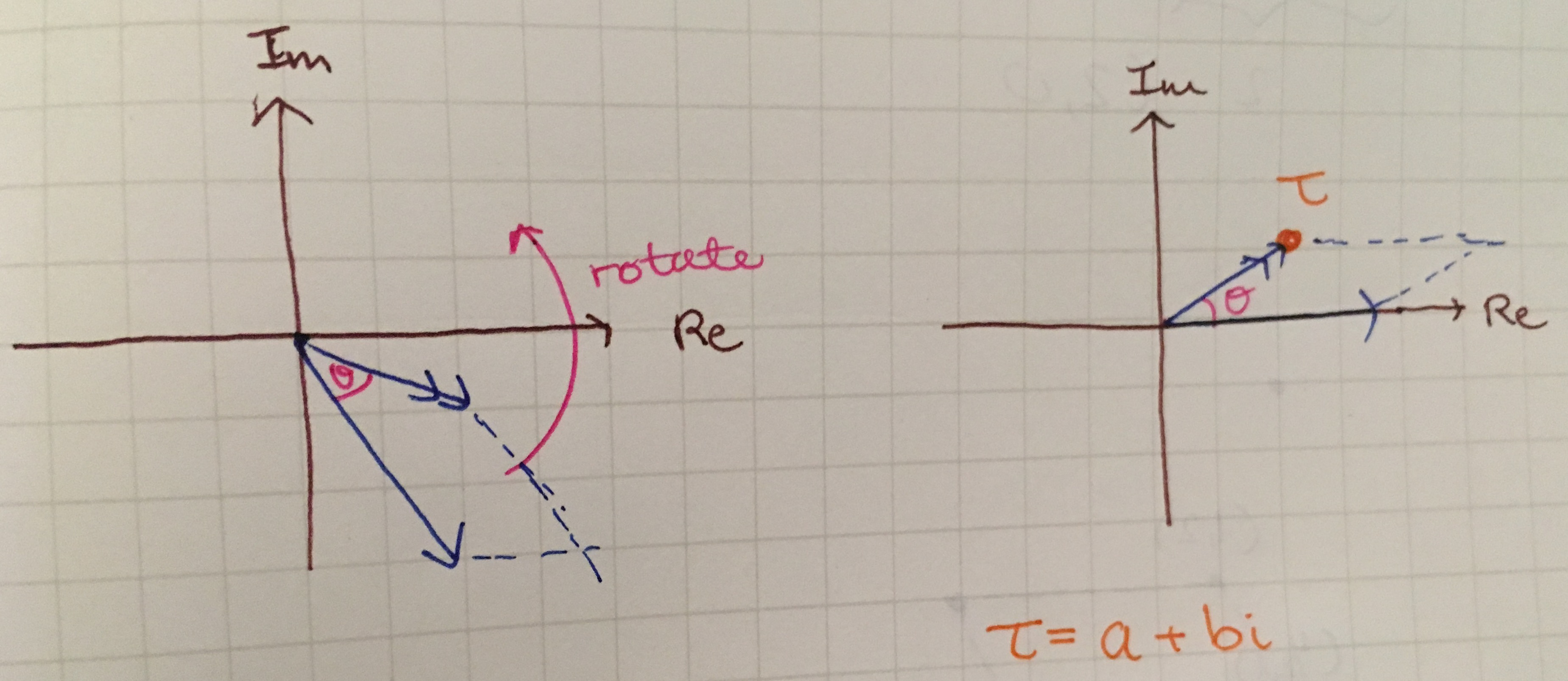We may represent our two-dimensional space as the complex plane. Then, then since the vector $v$ can always be rotated to be on the upper half-plane, we may uniquely identify our torus with the rotated coordinates of our vector $v = \tau = a + bi,$ assuming the torus has area $1.$

The group action of $\text{SL}_2(\mathbb{R})$ on the image of the lattice becomes $\text{SL}_2(\mathbb{R})/O(2)$ where $O(2)$ is the group of two-dimensional rotation.

Due to our equivalence of parallelograms being up to rotation and scale, we can fix our first basis vector to be of length $1$ lying on the real-axis. We may then uniquely identify each representative of our equivalence class by the coordinates of the second basis vector, which we will refer to as the parameter $\tau = a + bi$ in the complex upper half-plane.

Possible point of confusion: Are irrational coordinates allowed?

Irrational coordinates are allowed by definition. The domain of the $\text{SL}_2(\mathbb{Z})$ action should not be confused with that of $\text{SL}_2(\mathbb{R})$.

## The Teichmüller space of the torus

The space of possible coordinates of $\tau$ which uniquely identifies a torus under our new equivalence is exactly the Teichmüller space of the torus. Thus $\tau(T^2) = \mathbb{H}$, the complex upper half-plane.

Note: From the identification of the complex upper half-plane to this (Teichmüller) space of tori, we inherit the hyperbolic metric $ds = \lvert{dz}\rvert/\Im z$, which gives a useful notion of distance between tori in the Teichmüller space (for reasons not explored here).

But how do we make sense of that? In the definition of the Teichmüller space, there was mention of distinguished curves and markings, and of mapping class group action…

This concludes the first part of this post (yay! We did it!). In the second part, we will explore markings of the torus, the mapping class group and tie all the previous concepts we explored together. If you were new to the material, this is a good break point.

## Markings on a torus

As seen previously, the maps which embed the bases ${(1,0), (0,1)}$ (in green) and ${(1,0), (1,1)}$ (in pink) have the same image, so one could consider them the same as maps. However, if we ‘mark’ the resulting torus by the curves they embed, they become different as marked tori.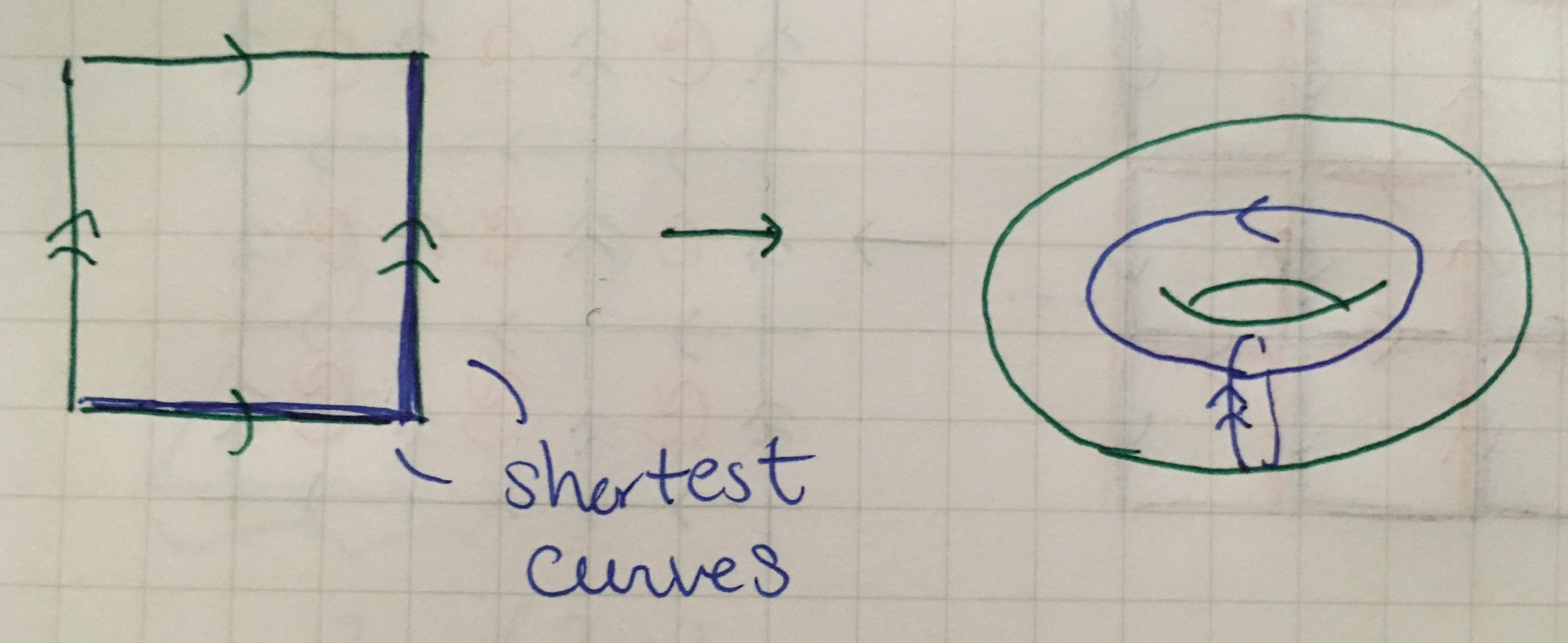Shortest curves in blue on the green torus, which both correspond with the generators of the fundamental group (the edges of the square).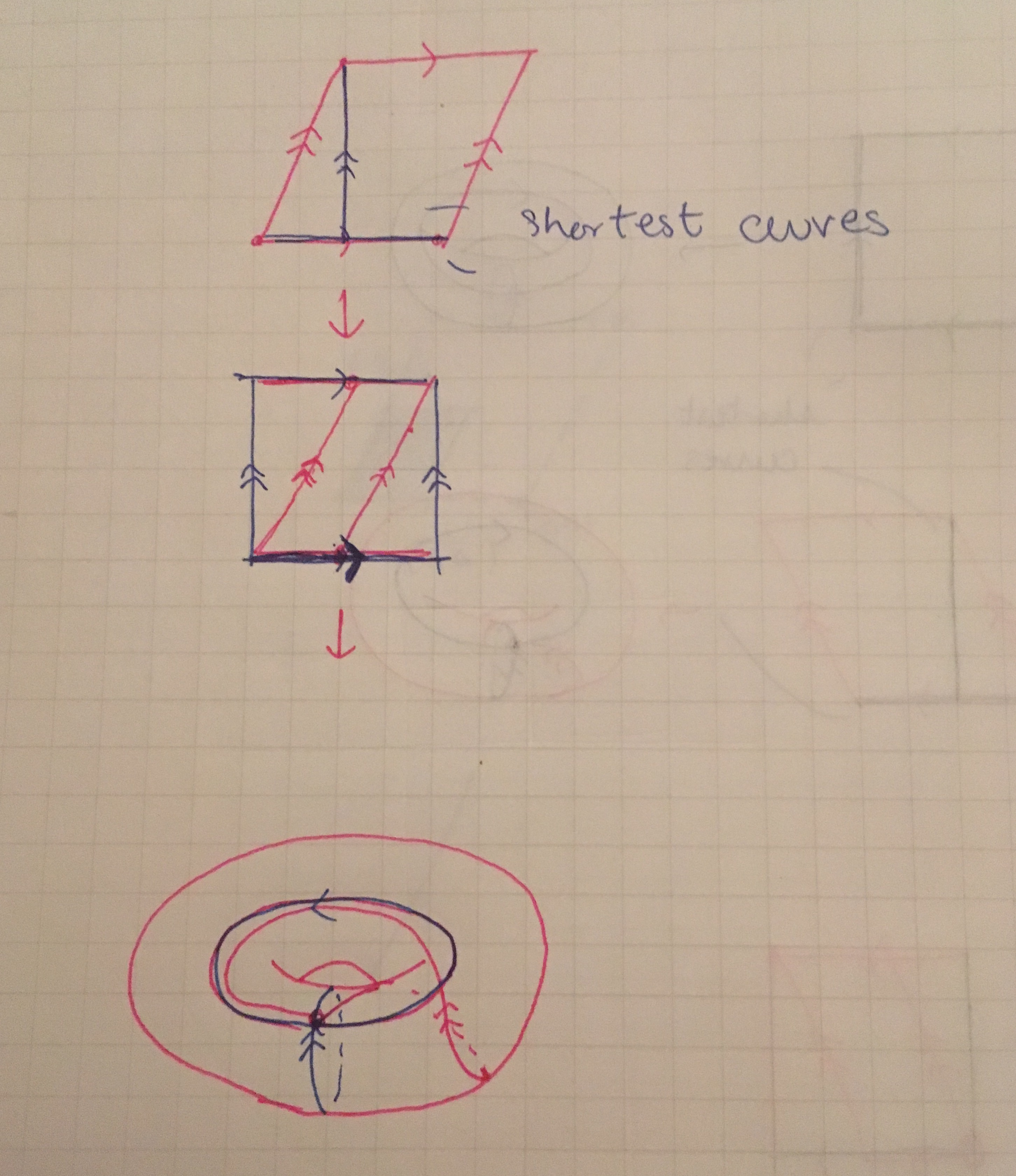Shortest curves in blue on the pink torus, where only one correspond with the generators of the fundamental group (the edges of the pink parallelogram).

Indeed, the green basis maps to the shortest curves on the torus, whereas the pink basis maps the second vector to a curve that is not the shortest.

A torus is fundamentally a product of two circles $S^1 \times S^1$. If you have a parallelogram with identified edges, you have infinitely many circles to choose from. The standard two circles (in blue) are supposed to represent the shortest two loops on any torus. As the blue curves illustrate, the shortest curves are not necessarily always the curves you pick to generate your torus (ex: pink torus).

Here are different interpretations of markings.

### Topological definition

Let $\gamma_1, \gamma_2$ be two circles, and let $S$ be the genus $1$ surfaces generated by $S = S^1 \times S^1 = \gamma_1 \times \gamma_2$.

In our examples, such an $S$ would be a fixed parallelogram $P$ in $\mathbb{Z}^2$ with the sides identified - let’s take $P$ to be a square with sides $1$ for simplicity. The horizontal sides of $P$ would correspond to $\gamma_1$ and the vertical sides to $\gamma_2$.

A marking of the torus is a orientation-preserving homeomorphism $f$ from $S$ to a Riemann surface $X$ where $f$ is fixed by once-intersecting simple closed curve $(\lambda_1, \lambda_2)$ on $X = \lambda_1 \times \lambda_2$ such that $f(\gamma_1) = \lambda_1, f(\gamma_2) = \lambda_2$.

In the pink torus example, $X$ is the pink torus and $(\lambda_1, \lambda_2)$ are the pink curves, and they are the (non-standard) generators the fundamental group $\pi_1(X)$. The blue curves represent the standard generators of $\pi_1(X)$. The map sending the parallelogram $P$ to the pink torus is the homeomorphism map $f$ from $S$ to $X$. The green torus example is similar, except that the green curves coincide with the blue curves because they are the standard generators of the fundamental group.

We say that $X$ and $Y$ are equivalent as markings if there is an isometry $\varphi$ between the homeomorphisms $f : S \to X$, $g : S \to Y$ such that $\varphi$ is isotopic (or homotopic) to the identity.

An example of such a homeomorphism isotopic to the identity is “introducing a wiggle” to a curve. Indeed, two curves as isotopic if they bound a disk. The proof relies on the “Alexander trick” on a disk, which can be generalized to prove isotopies of hyperbolic surfaces. (More in A Primer of Mapping Class Groups section 2.2.)

A non-example of a homeomorphism homotopic to the identity is a Dehn twist, which consists of cutting, rotating and gluing back.

The markings of green and pink tori differ because the pink marking has a Dehn twist. (Scroll up!)

Schwartz’s Mostly Surfaces Chapter 20 offers good coverage of this point of view.

### Group theoretic connection

We may consider different choices of curves representing the generators of the fundamental group as different markings of the torus.Note: emphasis on choice of curves, not the generators themselves.

Let $(X_1, \lambda_1, \lambda_2)$ be the green torus with green distinguished curves and $(X_2, \Lambda_1, \Lambda_2)$ the pink one with pink curves. Then $\pi_1(X_1) = \pi_1(X_2) = \langle a, b \mid ab = ba \rangle$. For the green torus, we have picked $\lambda_1 = a, \lambda_2 = b$ (the distinguished curves are the standard elements of the fundamental group as we expect). For the pink torus, we pick the pink curves to represent$\Lambda_1 = ba = a + b$, $\Lambda_2 = b$.

If we go back to thinking of the marked tori as parallelograms in $\mathbb{R}^2$ and then are markings as different choices of bases of $\mathbb{R}^2$ which we identify with arrows.

### Interesting combinational fact

Let $X$ be the torus identified with the distinguished curves $\alpha, \beta$ of rational slope $p/q, r/s$. In general, slopes need not be rational as the Teichmüller space admits irrational coordinates. But let’s stick to rational slopes for the sake of the interesting result that will follow.

We may re-shape the torus such that the distinguished curves lie at the center of the square-looking torus.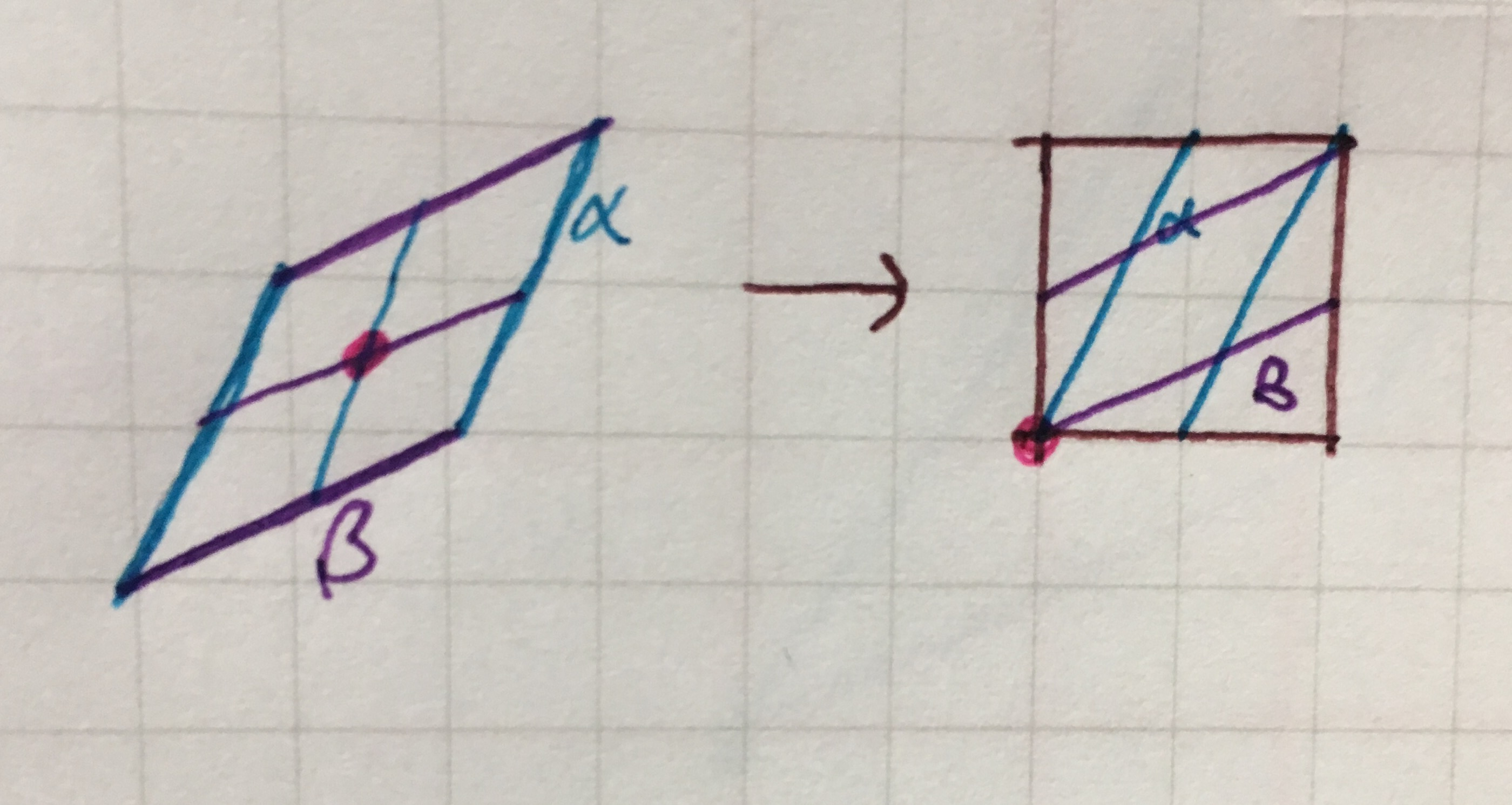In this example, $\text{slope}(\alpha) = 2/1, \text{slope}(\beta) = 2/1$.

Then, we may lift torus to its universal cover $\mathbb{R}^2$ along with the distinguished curves, such that a unit square is a copy of the square torus, and the distinguished curves become segments from $(0,0)$ to $(q,p)$ and $(r,s)$ respectively.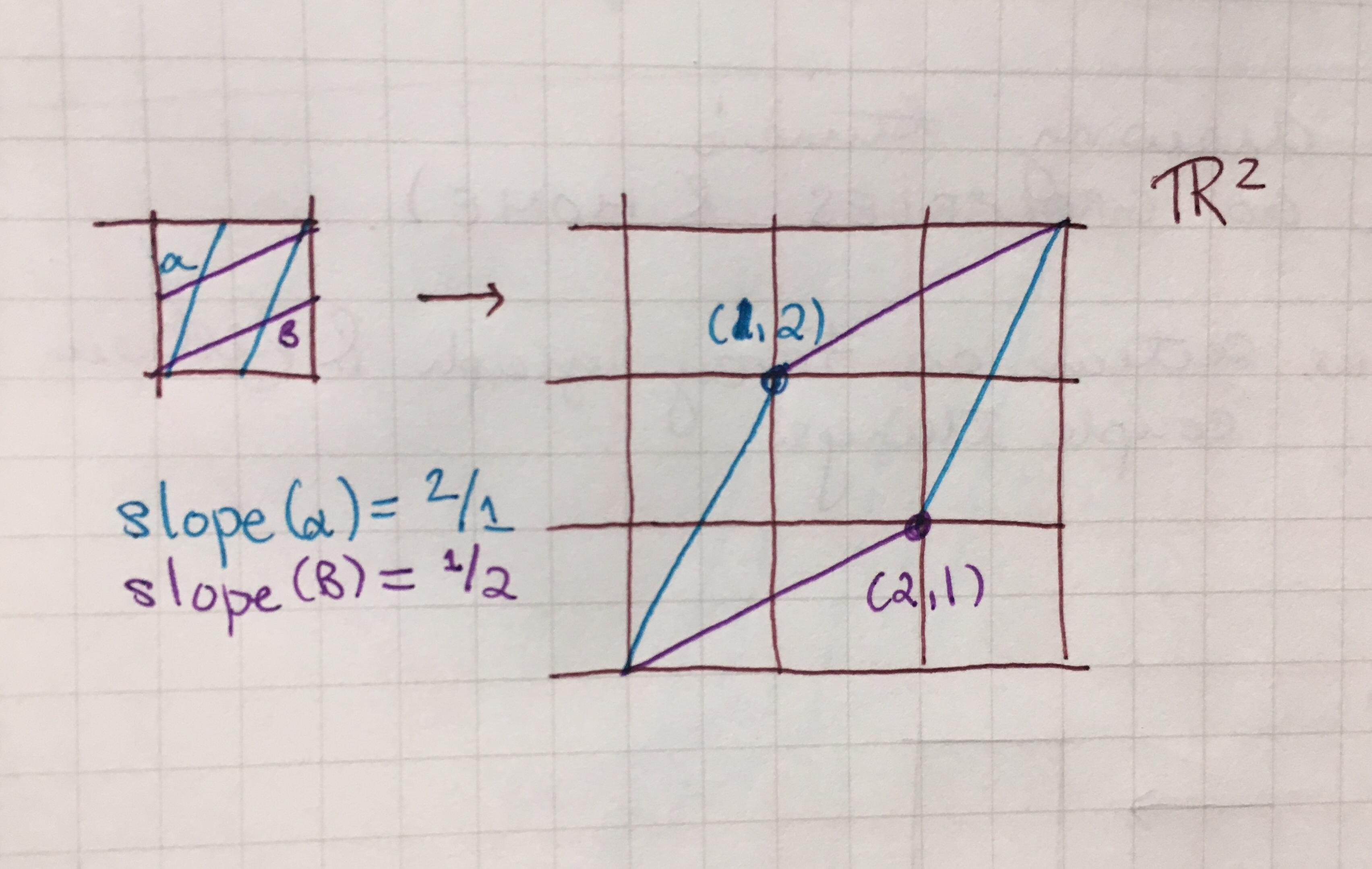In this example, $(q,p) = (1,2), (r,s) = (2, 1).$

We are interested in knowing how many lattice points there are on the on the curves $\alpha, \beta$, as these correspond to the number of intersection points $i(\alpha, \beta)$. The interior lattice points biject to the intersection points via redrawing the distinguished slopes (see below)

In our example, the number of intersection point is clearly $3$ by inspection.

Using Pick’s theorem, the area of a parellogram is given by $A = j + \frac{b}{2} - 1$ where $j$ is the number of interior points and $b$ is the number of points on the boundary. Some interior points, will biject (from our previous correspondance) to points on the boundary. Since we don’t want to double count for degenerate cases, we say that there are $4$ points on the boundary which are the $4$ corners of the parallelogram – the rest will be counted by $j$.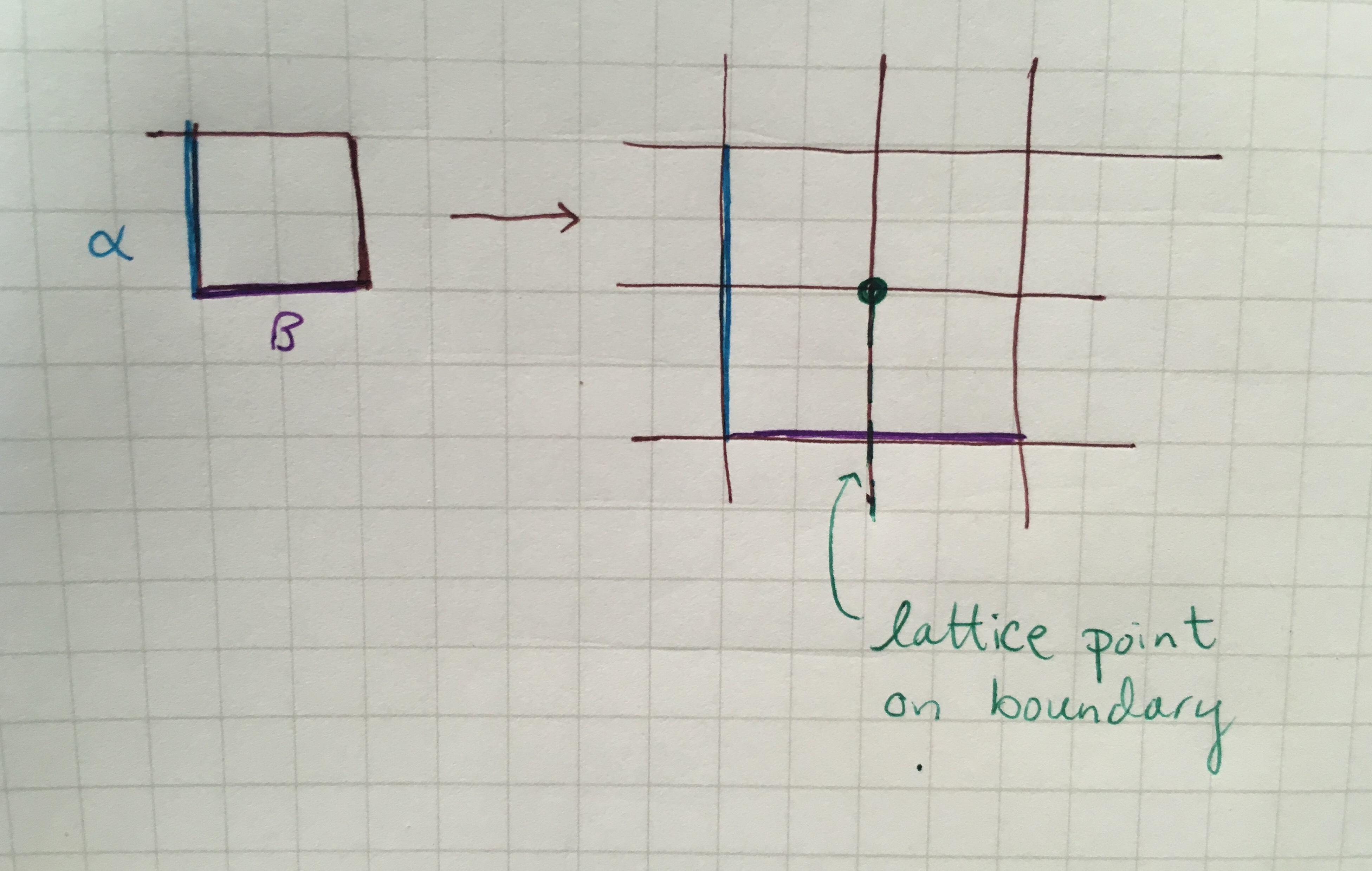Example of double counting of points on the boundary. The lift of $\beta$ has a lattice point on its at (1,0), which is already counted by the green interior point.

Thus, the number of intersection points in the interior of the lattice is given by

\begin{align*} & j = A - \frac{b}{2} + 1 \\ & j = \det(P) - \frac{4}{2} + 1 \\ & j = \left|rq - ps\right| - 1 \end{align*} Now, $j + 1 = i(\alpha, \beta)$, since the number of intersections is one plus the number of interior points by the argument above. Thus

This number is also known as the algebraic intersection of the torus.algebraic as opposed to geometric. Algebraic intersection numbers are oriented whereas geometric intersection numbers are not.

Punchline A torus has intersection number $1$ if and only if it’s determinant is $1$!

## Mapping Class Group of the Torus

The Mapping class group of the torus is $\text{SL}_2(\mathbb{Z})$ . It is well-known that $\text{SL}_2(\mathbb{Z})$ are generated by the elementsNot that I knew lol. Prof. Rafi subsequently remarked that the operations which do not change the determinant are adding one row with one another, and switching two rows, which is exactly what these two matrices represent. Now we know :)!

This is the change of basis group for $\mathbb{Z}^2$ that we have hinted at at the beginning. It has a natural action on the Teichmüller space, as we will see in the next section.

For now, we are going to offer a few interpretations of the action of the mapping class group as relating to the torus itself.

Note: Computing the mapping class group. Roughly speaking, the mapping class group can be computed using a variant of the Alexander method on the annulus. We are not going to do this here. Farb and Margalit do this in Primer to Mapping Class Groups section 2.2. There will be more references on this at the end.

### Group Theoretic

Each element of the mapping class group takes the standard basis to a to unique basis of $\mathbb{Z}^2$. Equivalently, the mapping class group acts faithfully on the isomorphism maps between $\pi_1(S) \to \pi_1(X)$.

### Topological

The action of the map is given by natural the composition of the homeomorphism $h$ on the homeomorphisms given by the markings.

$A$ corresponds to making a Dehn twist on the second generator,

whereas the $B$ interchanges the markings and reverses the direction of one of them.

At first, these maps can seem odd and difficult to picture as homeomorphisms compared to our vanilla interpretations of homeomorphism (at least in the homotop sense which we are used to when we picture a cup changing into a donut), and made me doubt they were actual homeomorphismsso continuous and bijective but then we realize that’s because the mapping class group is exactly the homeomorphisms which are not isotopic to the identity!

Then, I remembered the torus vs its embedding in three-space. Viewing the tori as what they actually are, which is the cross-section of two circles, the continuity and bijectivity of the maps are clearer.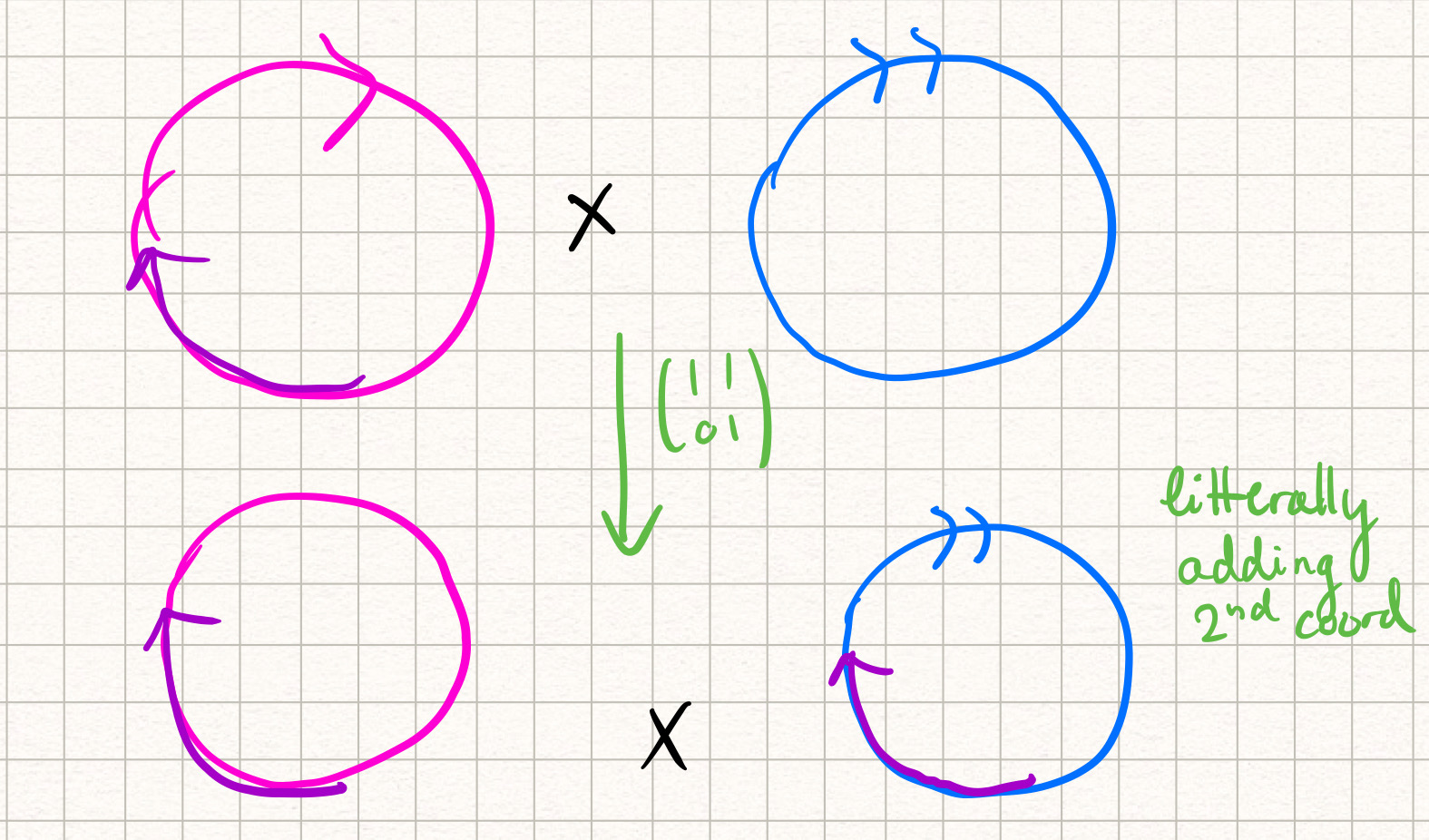This map is continuous since every small variation in its image is induced by a small variation in its domain. It is bijective since it has an inverse, which is marking an arc in the opposite direction on the second coordinate. Note that this picture is misleading since the domain is over integers, not continuous circles. Thus, there are no arcs that don't form a loop such as the purple one (that is drawn for intuition).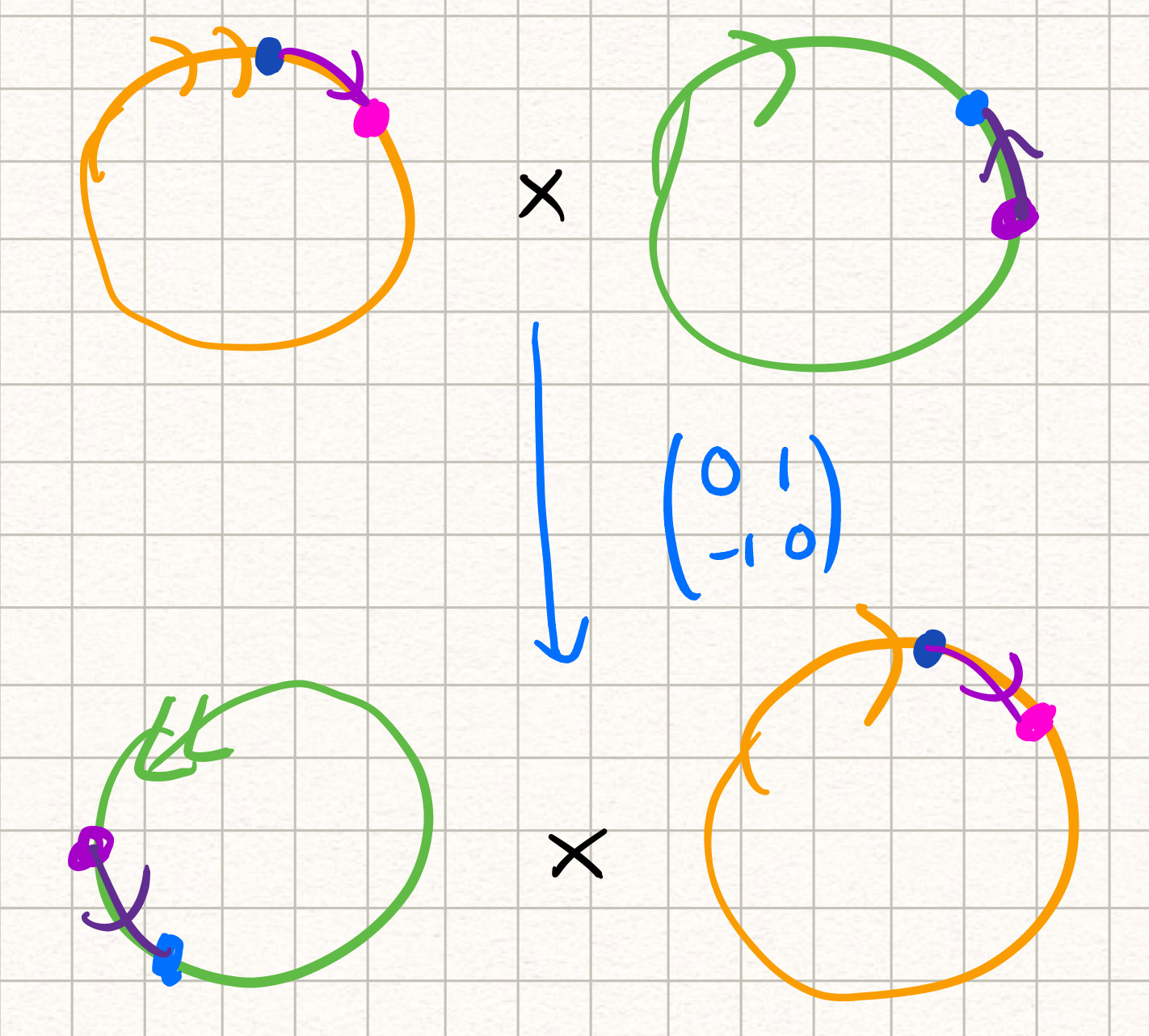### Combinatorial

(Nice connection with Farey graph)

Perhaps the most beautiful connection,

$\mid rq - ps \mid = 1 \iff \frac{p}{q}, \frac{r}{s}$ form an edge on the Farey graph. $\text{SL}_2(\mathbb{Z})$ acts on the graph in the following way.

which we interpret as

respectively.

The first generators sends the edge $(1/0, 0/1) \mapsto (1/1, 0/1) \mapsto (1/2, 0/1) \mapsto (1/3, 0/1)$ and so on. The second generator inverts the direction of the edge.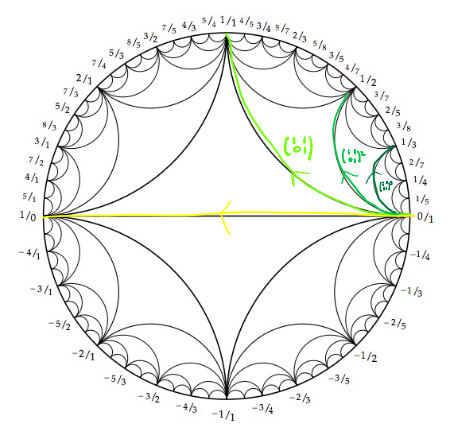Picture illustrating the action of the MCG on the Farey graph. The orientation of the arrow indicates which vertex stays fixed at each step.

If we applied the second generator we would start with $(0/1, -1/0) \mapsto (-1/1, -1/0) \mapsto (-2/1, -1/0) \mapsto (-3/1, -1/0)$ and so on.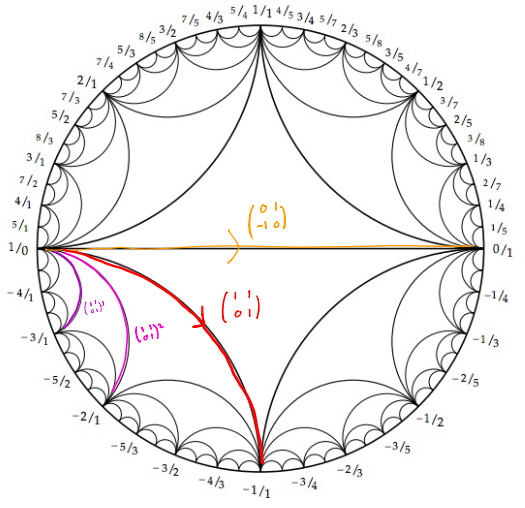Picture illustrating the action of the MCG on the Farey graph. The orientation of the arrow indicates which vertex stays fixed at each step.

This is beautifully illustrated on the Farey graph, where $1/0 = -1/0 = \infty$.

$\begin{pmatrix} 1 & 1 \ 0 & 1 \end{pmatrix}^{-1}$ sends $(1/0, 0/1) \mapsto (-1/1, 0/1)$ $\mapsto (-1/2, 0/1) \mapsto (-1/3, 0/1).$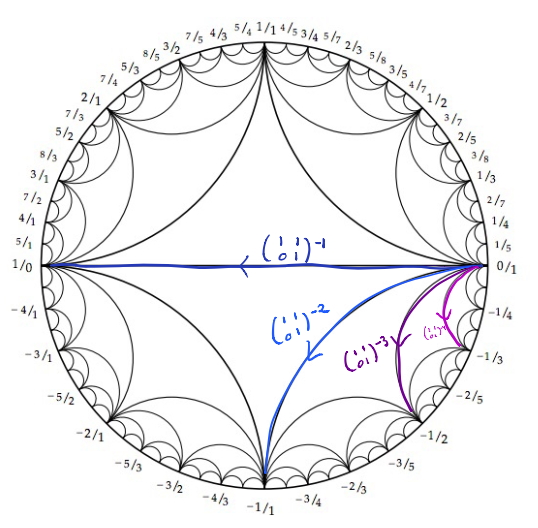Picture illustrating the action of the MCG on the Farey graph. The orientation of the arrow indicates which vertex stays fixed at each step.

With these pictures, it is easy to see that the action of the mapping class group is transitive on the Farey graph.

Possible point of confusion: Are irrational slopes not markings since they are elements of the Teichmuller space? Shouldn’t the mapping class group act transitively on those too?

I was confused about this for a while. Yes, the irrational slopes are also markings since they have representative in $\mathbb{H}$, which is the space of markings. They do not correspond to an element of the mapping class group in the sense there is no bijection between an element of the MCG and an irrational basis in the edges of the Farey graph like there is for rational bases (by sending $p/q$ to the element mapping $0 \mapsto p/q$.)

Indeed, the mapping class group has a natural action on the Teichmüller space (say by adding a Dehn twist to a marking or inverting the markings), but its definition is first and foremost a topological definition relating to classes of orientation-preserving homeomorphisms. It’s very easy to confuse the mapping class group with the group permuting the markings, but it is not. The group acting transitively the markings for the torus is $\text{PSL}_2(\mathbb{R})$,while the mapping class group is $\text{SL}_2(\mathbb{Z})$, which does have a natural $\text{PSL}_2(\mathbb{Z})$ action on the Teichmüller space, as we will see in the last section.

### Note on Moduli Spaces

You may be wondering what’s left of $\mathbb{H}$** once we mod by the action of $\text{SL}_2(\mathbb{R})$. That is precisely what the moduli space of the torus is.The moduli space of the torus is given by the Teichmüller space modulo the action of the mapping class group.

The tori with basis ${(1,0), (0,1}$ and ${(1,0),(1,1)}$ we saw at the beginning have different $\tau$ parameters ($i$ and $i+1$ respectively) and thus are distinct as points in the Teichmüller space. However, they represent the same point in the moduli space of the torus because they differ by a Dehn twist.

To come back to the previous possible point of confusion, if the MCG acted transitively on the Teichmüller, then the moduli space would be trivial. Instead, it is represented by the fundamental domain of $\text{SL}_2(\mathbb{Z})$ over the $\mathbb{H}$.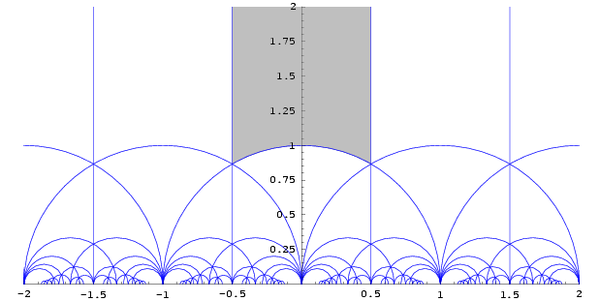In grey: the fundamental domain representing the moduli space of the torus. In blue: planar version of the Farey graph bouding free regular sets. Read more in source: [wikipedia](https://en.wikipedia.org/wiki/Fundamental_domain).

## Putting it all together

Here comes the last punchline. Recall from complex analysis that $\text{PSL}_2(\mathbb{R})$ acts isometrically transitively on the upper-half plane $\mathbb{H}$If you can’t recall this, that’s totally fine - it’s a fact of complex analysis that should be available in any textbook :) , which can be thought of as the Teichmüller space of the torus. An element of $\text{PSL}_2(\mathbb{R})$ is of the form

and acts by Mobius transformation on an element $\tau \in \mathbb{H}$

The elements of $\text{SL}_2(\mathbb{Z})$ are of the same form as those of $\text{PSL}_2(\mathbb{Z})$, but we picked its action to be linear over the bases of $\mathbb{Z}^2$ (change of basis action).

Thus, there is a map from the mapping class group $\text{SL}_2(\mathbb{Z})$ of the torus to the Mobius transformation $\text{PSL}_2(\mathbb{R})$ of the upper-half plane, and this group turns out to be the group of isometries in the hyperbolic plane $\mathbb{H}$.

Recall the generators for $\text{SL}_2(\mathbb{Z})$ and $\text{PSL}_2(\mathbb{Z}) = \text{SL}_2(\mathbb{Z})/\langle \pm 1 \rangle$Meaning $A, -A \in \text{SL}_2(\mathbb{Z})$ represent the same element in $\text{PSL}_2(\mathbb{Z})$. This is because the minus sign cancel on the top and bottom of the fraction (write it out if you do not see it)! :

$A$ sends by linear fractional transformation the point $\tau$ on the upper half-plane to $\frac{1 \cdot \tau + 1}{0 \cdot \tau + 1} = \tau + 1$. $B$ sends $\tau \mapsto \frac{0 \cdot \tau + 1}{-1\cdot \tau + 0} = - \frac{1}{\tau}$.

Take your standard basis torus generated by ${(1,0),(0,1)}.$ This is identified with the point $i \in \mathbb{H}$.

Under the $\text{PSL}_2(\mathbb{Z})$ action of $A$, $i$ gets sent to $i + 1$, which corresponds to the torus generated by ${(1,0),(1,1)}$. But this is also exactly where the linear $\text{SL}_2(\mathbb{Z})$ action of $A$ sends our standard basis!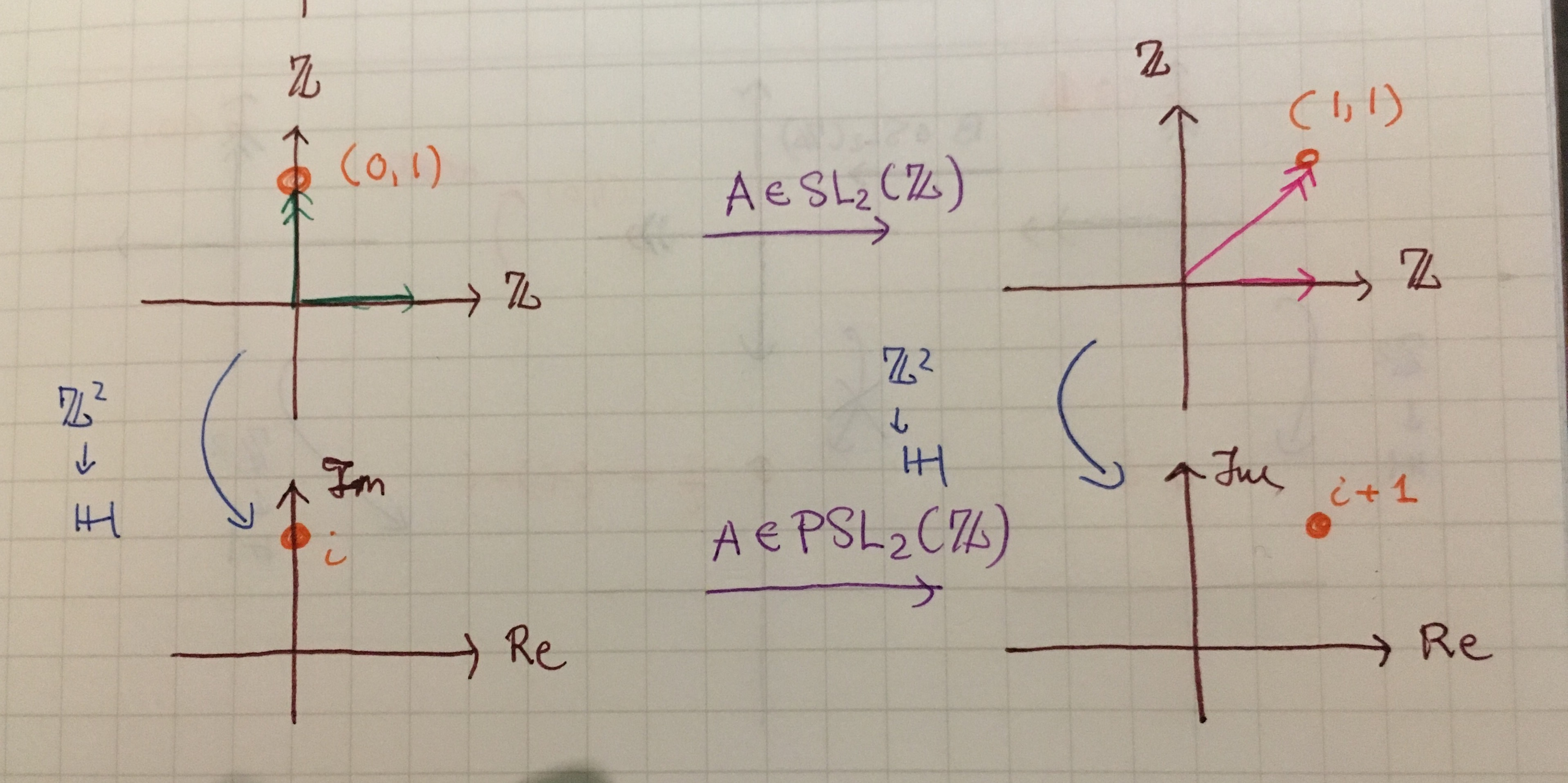Shortest curves in blue on the pink torus, where only one correspond with the generators of the fundamental group (the edges of the pink parallelogram)

Under the $\text{PSL}_2(\mathbb{Z})$ action of $B$, $i$ gets sent to $-\frac{1}{i} = - (-i) = i$. This is also exactly where the linear $\text{SL}_2(\mathbb{Z})$ action of $A$ sends our standard basis once we’ve rotated the image back to the upper half-plane.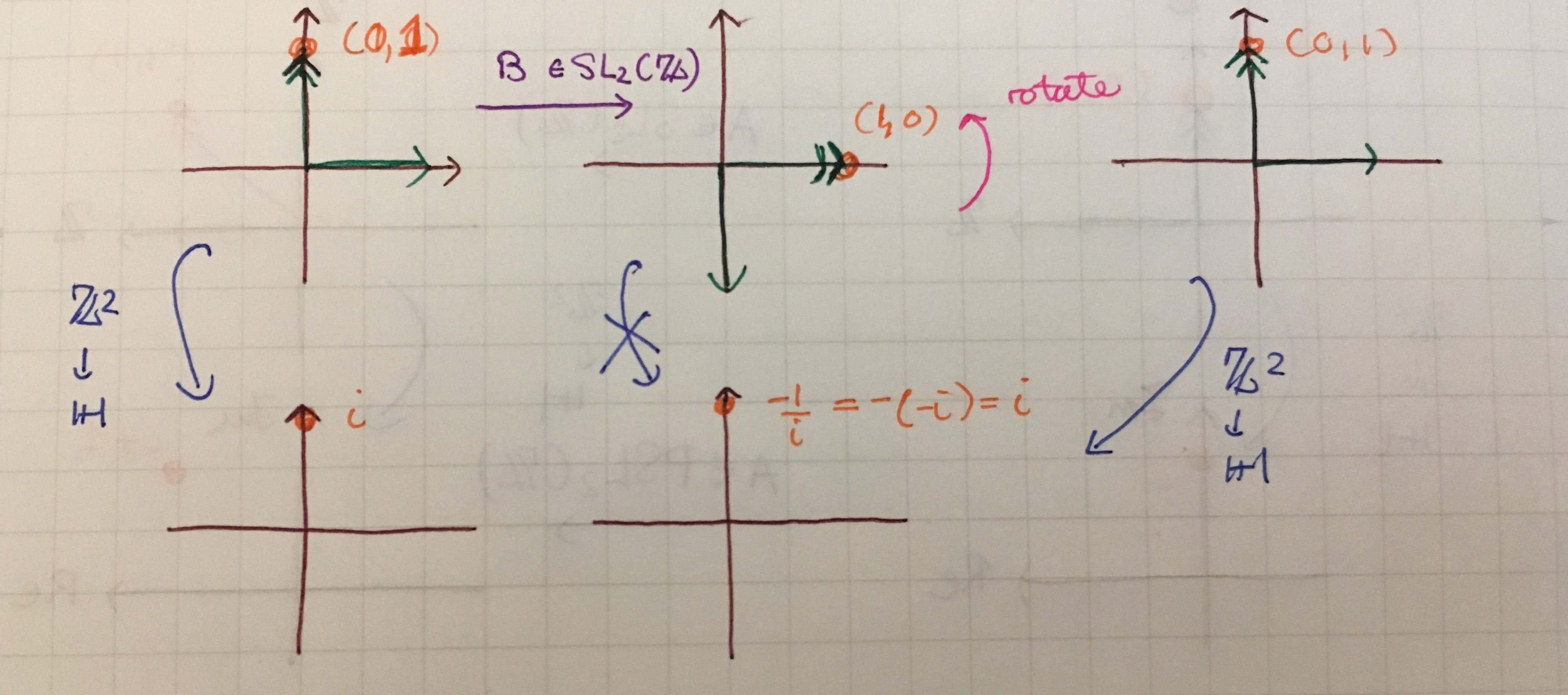Shortest curves in blue on the pink torus, where only one correspond with the generators of the fundamental group (the edges of the pink parallelogram)

The action of the Mobius transformation on the complex plane is compatible with the action of $\text{SL}_2(\mathbb{Z})$ on the the lattice!

To Prof. Rafi, there is more to this than pure coincidence. This is one of the reason why we study mapping class groups, Teichmüller spaces and hyperbolic surfaces as as a trifecta of some sort!

Ouf! We are done. Give yourself a big tap on the back :).

Hope you enjoyed this, and that everything makes much more sense when you reread the definitions!

## Sources and Resources

Kasra Rafi as recalled by me. Errors are mine. Please let me know of them!

Notes by Evan Schwarz Chapter 20.

A Primer on Mapping Class Groups by Farb and Margalit, sections 10.2 and 10.3; 2.2 for the Alexander method and the computation of the mapping class group of the torus. Here are illustrated textbook pages on that section (illustrations mine).

## Acknowledgements

Thanks to Kasra Rafi for his time and patience teaching this and for agreeing to proofread this!

Thanks to Felix Bauckholt (currently an undergrad at Waterloo), for his great questions and feedback which allowed me to significantly clarify this blog post. Always a pleasure to discuss math with you!

## Get in touch

I’m looking for people to read drafts of my blog posts and have 1-1 discussions with. If you’re a motivated, supportiveVery important to me. I don’t know everything, and sometimes don’t know anything. undergrad, grad student, or other qualified person who wants to read drafts of my blog posts, ask clarifying questions and give me feedback in return for some 1-1 discussion of hyperbolic geometry, get in touch with me!

Intro to Mapping Class Groups, Teichmuller Spaces and Hyperbolic Surfaces. - October 8, 2017 - Hang Lu Su